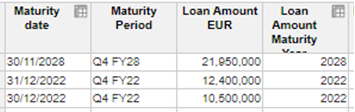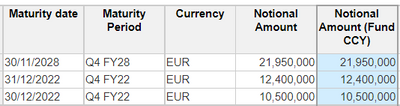# Summing on Period (Quarter)Hi,

I need some help in summing on the maturity period (quarters).

Module 1: no time dimension, standing data showing loan amounts in various currencies, and maturities.Module 2 is dimensioned by time (quarters) and the loan amounts are converted at the quarterly FX rates.Module 3 is dimensioned by time (quarters) and I want to create a module that shows when the loans mature.  How do I go about doing this?
I've tried summing on the converted loan amounts in Module 2 which does not work. Summing on the standing data allocates the loans to the correct maturity period, but the loans are of various currencies and need to be converted into EUR.

Thanks,

Tagged:

•Hello,

If I have understood this correctly, I think you should be able to achieve this in Module 2.

I set up the following modules:

Module 1 with the standing data not dimensioned by time

Module 2 with the currency values

I added the line item to test the item quarter against the maturity period

Which allows you to pivot the report by time to see the values that mature by quarter.

Regards

Bill

•Hello,
To create a module that shows when the loans mature, you can follow these steps:

1. In module 1, create a line item for the converted loan amounts in EUR. This line item should be dimensioned by currency and time (quarters).
2. In module 2, use a formula to convert the loan amounts from various currencies to EUR using the quarterly fx rates. You can use a lookup function or a mapping module to retrieve the fx rates for each currency and quarter.
3. In module 3, create a line item for the maturity period. This line item should be dimensioned by time (quarters).
4. Use a formula in module 3 to sum the converted loan amounts from module 2 based on the maturity period. You can use a SUM function with a filter to sum the loan amounts for each quarter.

Here's an example formula for the maturity period line item in module 3:
SUM(Module2.Converted Loan Amounts) WHERE Module2.Quarter = Maturity Period.Quarter

Make sure to adjust the module and line item names to match your specific model. This formula will sum the converted loan amounts for each quarter and allocate them to the correct maturity period.

Let me know if you have any further questions or need additional assistance!

Regards,
Sambhav